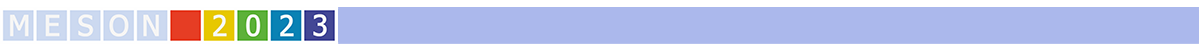#MESON2023

Jun 22 – 27, 2023
Auditorium Maximum
Europe/Warsaw timezone

## The $T_{c\bar{s}}(2900)$ as a threshold effect from the interaction of the $D^*K^*$, $D^*_s\rho$ channels

Jun 22, 2023, 5:35 PM
20m
Medium lecture hall (A) (Auditorium Maximum)

Parallel

### Speaker

Raquel Molina Peralta (IFIC)

### Description

We look at the mass distribution of the $D_s^+ \pi^-$ in the $B^0 \to \bar{D}^0 D_s^+ \pi^-$ decay, where a peak has been observed in the region of the $D^*_s \rho$, $D^* K^*$ thresholds. By creating these two channels together with a $\bar{D}^0$ in $B^0$ decay and letting them interact as coupled channels, we obtain a structure around their thresholds, short of producing a bound state, which leads to a peak in the $D_s^+ \pi^-$ mass distribution in the $B^0 \to \bar{D}^0 D_s^+ \pi^-$ decay. We conclude that the interaction between the $D^*K^*$ and $D^*_s\rho$ is essential to produce the cusp structure that we associate to the recently seen $T_{c\bar{s}}(2900)$, and that its experimental width is mainly due to the decay width of the $\rho$ meson. The peak obtained together with a smooth background reproduces fairly well the experimental mass distribution observed in the $B_0 \to \bar{D}^0 D_s^+ \pi^-$ decay.

### Primary author

Raquel Molina Peralta (IFIC)

### Co-author

Eulogio Oset Baguena (IFIC)